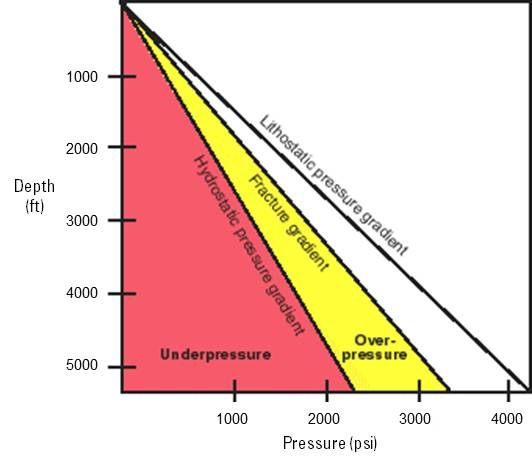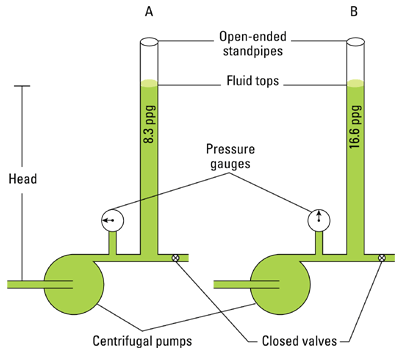# Explore the Energy Glossary

Look up terms beginning with:

# hydrostatic pressure

1. n. []

The normal, predicted pressure for a given depth, or the pressure exerted per unit area by a column of freshwater from sea level to a given depth. Abnormally low pressure might occur in areas where fluids have been drained, such as a depleted hydrocarbon reservoir. Abnormally high pressure might occur in areas where burial of water-filled sediments by an impermeable sediment such as clay was so rapid that fluids could not escape and the pore pressure increased with deeper burial.2. n. []

The force per unit area caused by a column of fluid. In US oilfield units, this is calculated using the equation: P=MW*Depth*0.052, where MW is the drilling fluid density in pounds per gallon, Depth is the true vertical depth or "head" in feet, and 0.052 is a unit conversion factor chosen such that P results in units of pounds per square in. (psi).3. n. []

The pressure at any point in a column of fluid caused by the weight of fluid above that point. Controlling the hydrostatic pressure of a mud column is a critical part of mud engineering. Mud weight must be monitored and adjusted to always stay within the limits imposed by the drilling situation. Sufficient hydrostatic pressure (mud weight) is necessary to prevent an influx of fluids from downhole, but excessive pressure must also be avoided to prevent creation of hydraulic fractures in the formation, which would cause lost circulation. Hydrostatic pressure is calculated from mud weight and true vertical depth as follows:
Hydrostatic pressure, psi = 0.052 x Mud Weight, lbm/gal x True Vertical Depth, ft. (To convert to SI units, 1.0 psi = 6.9 kPa.)